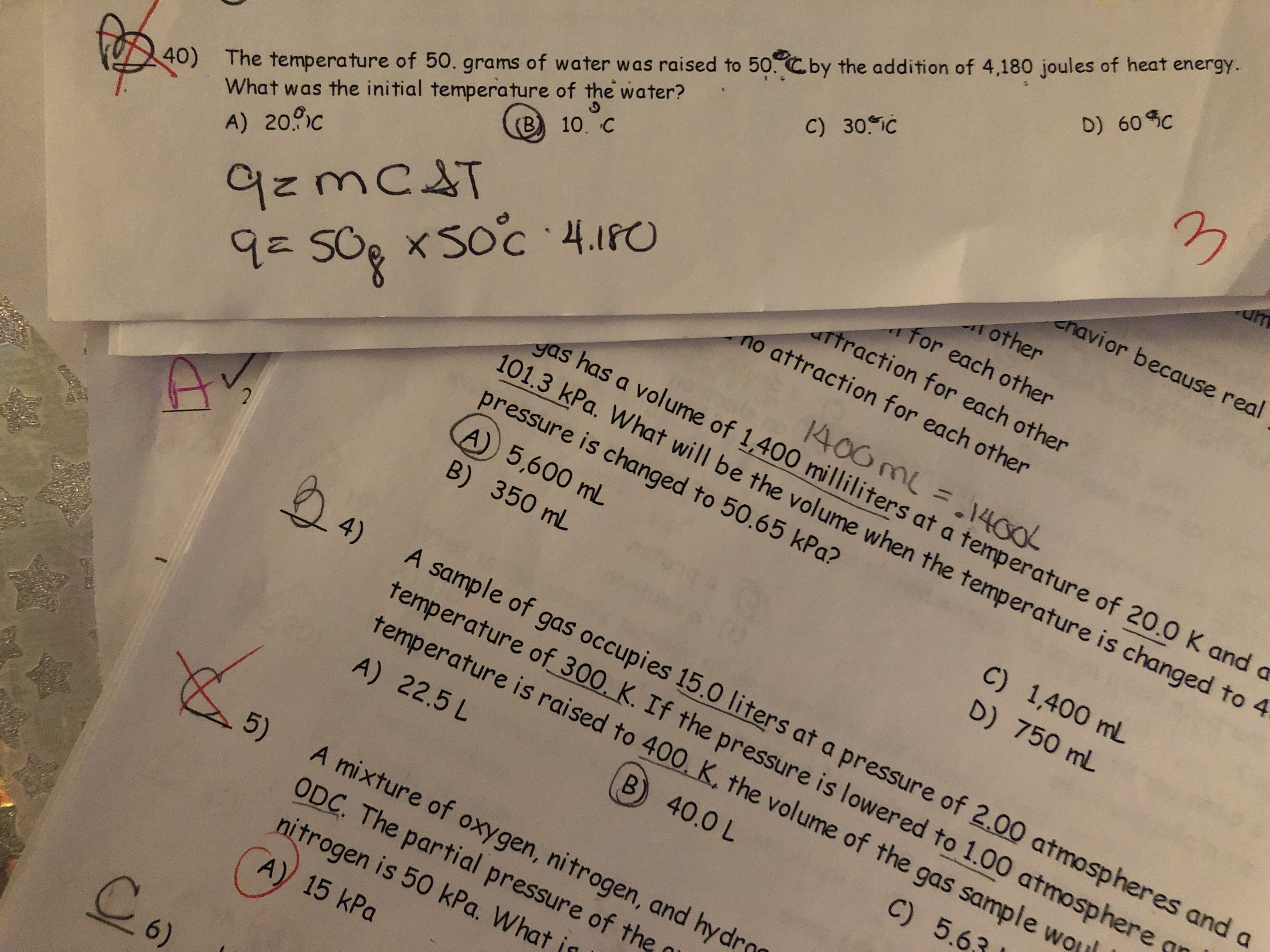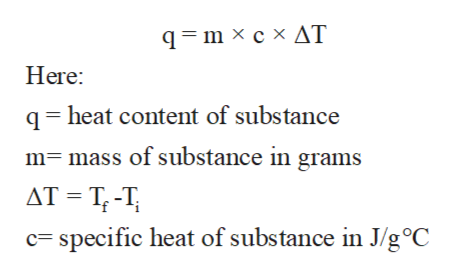# The temperature of 50. grams of water was raised to 50.Cby the addition of 4,180 joules of heat energy.What was the initial temperature of the water?D) 60 CC) 30. IC(B) 10. Ccnavior because real9zmC&T9=50g x Soc 4.1809= 50g xotherfor each otherartraction for each otherno attraction for each other40)A) 20.c1400 mL = .1400yas has a volume of 1,400 milliliters at a temperature of 20.0 K and a101.3 kPa. What will be the volume when the temperature is changed to 4pressure is changed to 50.65 kPa?A) 5,600 mLB) 350 mLC) 1,400 mLD) 750 mL24)A sample of gas occupies 15.0 Iliters at a pressure of 2.00 atmospheres and atemperature of_300. K. If the pressure is lowered to 1.00 atmospheretemperature is raised to 400, K, the volume of the gas sample woA) 22.5 LB) 40.0 LC) 5.6A mixture of oxygen, nitrogen, and hyd5)ODC. The partial pressure of thenitrogen is 50 kPa. WhatA) 15 kPaCo6)

Question
25 views

Question 40help_outlineImage TranscriptioncloseThe temperature of 50. grams of water was raised to 50.Cby the addition of 4,180 joules of heat energy. What was the initial temperature of the water? D) 60 C C) 30. IC (B) 10. C cnavior because real 9zmC&T 9=50g x Soc 4.180 9= 50g x other for each other artraction for each other no attraction for each other 40) A) 20.c 1400 mL = .1400 yas has a volume of 1,400 milliliters at a temperature of 20.0 K and a 101.3 kPa. What will be the volume when the temperature is changed to 4 pressure is changed to 50.65 kPa? A) 5,600 mL B) 350 mL C) 1,400 mL D) 750 mL 24) A sample of gas occupies 15.0 Iliters at a pressure of 2.00 atmospheres and a temperature of_300. K. If the pressure is lowered to 1.00 atmosphere temperature is raised to 400, K, the volume of the gas sample wo A) 22.5 L B) 40.0 L C) 5.6 A mixture of oxygen, nitrogen, and hyd 5) ODC. The partial pressure of the nitrogen is 50 kPa. What A) 15 kPa Co 6) fullscreen
check_circle

Step 1

Thermodynamic is the branch of chemistry that mainly deals with the heat transfer between system and surroundings. Here system is the substance for which the heat content is studied and remaining part of universe is consider as the surroundings.

Step 2

The heat content of any substance can be expressed in terms of heat capacity or specific heat. Heat capacity can be defined as the amount of heat to be supplied to a given mass of substance to produce a unit change in its temperature.

Step 3

Similarly the specific heat can be defined as the amount of heat per unit mass required to raise the temperature by 1 ◦C.

The specific heat of the sub...help_outlineImage Transcriptioncloseq=m × c × AT Here: q= heat content of substance m= mass of substance in grams AT = T; -T; c= specific heat of substance in J/g°C fullscreen

### Want to see the full answer?

See Solution

#### Want to see this answer and more?

Solutions are written by subject experts who are available 24/7. Questions are typically answered within 1 hour.*

See Solution
*Response times may vary by subject and question.
Tagged in

### Chemical Thermodynamics